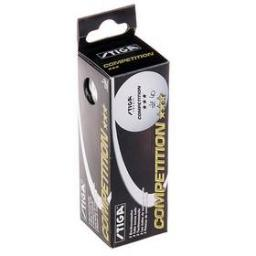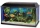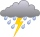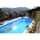# Balls

Ping pong balls have a diameter of approximately 5.1 cm. They are sold in boxes of 10 pieces: each box has the shape of a cuboid with a square base. The balls touch the walls of the box.

Calculate what portion of the internal volume of the box is filled with balls.

Result

x =  52.36 %

#### Solution:Our examples were largely sent or created by pupils and students themselves. Therefore, we would be pleased if you could send us any errors you found, spelling mistakes, or rephasing the example. Thank you!

Leave us a comment of this math problem and its solution (i.e. if it is still somewhat unclear...):

Showing 1 comment:UnkownTips to related online calculators
Check out our ratio calculator.
Tip: Our volume units converter will help you with the conversion of volume units.

## Next similar math problems:

1. AquariumThe box-shaped aquarium is 40 cm high; the bottom has dimensions of 70 cm and 50 cm. Simon wanted to create an exciting environment for the fish, so he fixed three pillars to the bottom. They all have the shape of a cuboid with a square base. The base edge
2. AquariumI have an aquarium that is 100 cm long and 40 cm wide and 40 cm in height. We fill it with water. How much will it weigh?
3. PebbleThe aquarium with internal dimensions of the bottom 40 cm × 35 cm and a height of 30 cm is filled with two-thirds of water. Calculate how many millimeters the water level in the aquarium rises by dipping a pebble-shaped sphere with a diameter of 18 cm.
4. PoolHow many hl of water is in a cuboid pool (a = 25m, b = 8m) if the area of the wetted walls is 279.2 m2?
5. Uboid volumeCalculate the cuboid volume if the walls are 30cm², 35cm², 42cm²
6. Swimming poolThe swimming pool has the shape of a block with dimensions of 70dm, 25m, 200cm. How many hl of water can fit into the pool?
7. Body diagonalCalculate the volume of a cuboid whose body diagonal u is equal to 6.1 cm. Rectangular base has dimensions of 3.2 cm and 2.4 cm
8. The cuboidThe cuboid is filled to the brim with water. The external dimensions are 95 cm, 120 cm, and 60 cm. The thickness of all walls and the bottom is 5 cm. How many liters of water fit into the cuboid?
9. Alien shipThe alien ship has the shape of a sphere with a radius of r = 3000m, and its crew needs the ship to carry the collected research material in a cuboid box with a square base. Determine the length of the base and (and height h) so that the box has the large
10. Wood prismsHow many weight 25 prisms with dimensions 8x8x200 cm? 1 cubic meter of wood weighs 800 kg.
11. RainwaterThe garden area of 800 square meters fell 3mm of rainwater. How many 10 liters of water can we water this garden equally?
12. The glass1 m3 of glass weighs 2600 kg. Calculate the weight of the glass glazing panel with dimensions of 2.5 m and 3.8 m if the thickness of the glass is 0.8 cm
13. Three-quarters of its volumeThe pool has a block shape with a length of 8m, a width of 5.3m and a depth of 1.5m. How many hl of water is in it if it is filled to three-quarters of its volume?
14. IcebergWhat is the surface area of 50 cm iceberg (in the shape of a cuboid) that can carry a man with luggage with a total weight of 120 kg?
15. Water tankWhat is the height of the cuboid-shaped tank with the bottom dimensions of 80 cm and 50 cm if the 480 liters of water reaches 10 cm below the top?
16. Prism 4 sidesFind the surface area and volume four-sided prism high 10cm if its base is a rectangle measuring 8 cm and 1.2dm
17. AquariumCan 30 liters of water fit in a cuboid aquarium with dimensions a = 3dm b = 6dm c = 5dm?